# The half life

The half-life of a radioactive isotope is the time it takes for a quantity of the isotope to be reduced to half its initial mass. Starting with 145 grams of a radioactive isotope, how much will be left after 3 half-lives?

m3 =  18.125 g

### Step-by-step explanation:Did you find an error or inaccuracy? Feel free to write us. Thank you!Tips to related online calculators
Do you want to convert time units like minutes to seconds?

## Related math problems and questions:A radioactive material loses 10% of its mass each year. What proportion will be left there after n=6 years?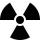After 548 hours decreases the activity of a radioactive substance to 1/9 of the initial value. What is the half-life of the substance?
• Half life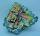Determine the half life of bismuth, when bismuth weight from the original weight of 32 g was only 2 grams in 242 minutes.
• CrystalThe crystal grows every month 1.2 permille of its mass. For how many months to grow a crystal from weight 177 g to 384 g?
• Suppose 2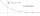Suppose that the half-life of a substance is 250 years. If there were initially 100 g of the substance, a. Give an exponential model for the situation b. How much will remain after 500 years?
• Geometric progressionIn geometric progression, a1 = 7, q = 5. Find the condition for n to sum first n members is sn≤217.
• The city 2Today lives 298000 citizens in the city. How many citizens can we expect in 8 years if their annual increase is 2.4%?
• DepositIf you deposit 719 euros at the beginning of each year, how much money we have at 1.3% (compound) interest after 9 years?
• The town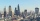The town population is 56000. It is decreasing by 2% every year. What will be the population of the town after 13 years?
• Virus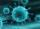We have a virus that lives one hour. Every half hour produce two child viruses. What will be the living population of the virus after 3.5 hours?
• Compound interestCompound interest: Clara deposited CZK 100,000 in the bank with an annual interest rate of 1.5%. Both money and interest remain deposited in the bank. How many CZK will be in the bank after 3 years?
• LoanApply for a \$ 59000 loan, the loan repayment period is 8 years, the interest rate 7%. How much should I pay for every month (or every year if paid yearly). Example is for practise geometric progression and/or periodic payment for an annuity.
• Three members GPThe sum of three numbers in GP (geometric progression) is 21 and the sum of their squares is 189. Find the numbers.
• Wire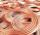One pull of wire is reduced its diameter by 14%. What will be the diameter of wire with diameter 19 mm over 10 pulls?
• Acid solutionBy adding 250 grams of a 96% sulfuric acid solution to its 3% solution, we changed its initial concentration to 25%. How many grams of 3% of the acid was used for dilution?
• Curiosity factor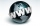A blogger starts a new website, initially the number of the traffic is 293 due to their curiosity factor. The business owner estimated that the traffic will increase by 2,6% per week. What will be the number of it in week 5?.
• The workThe work was to be done by 150 workers. At the beginning of their work, their number reduced by 40, which increased the time of work by 5 and 1/3 of the schedule. How long did work take?